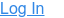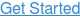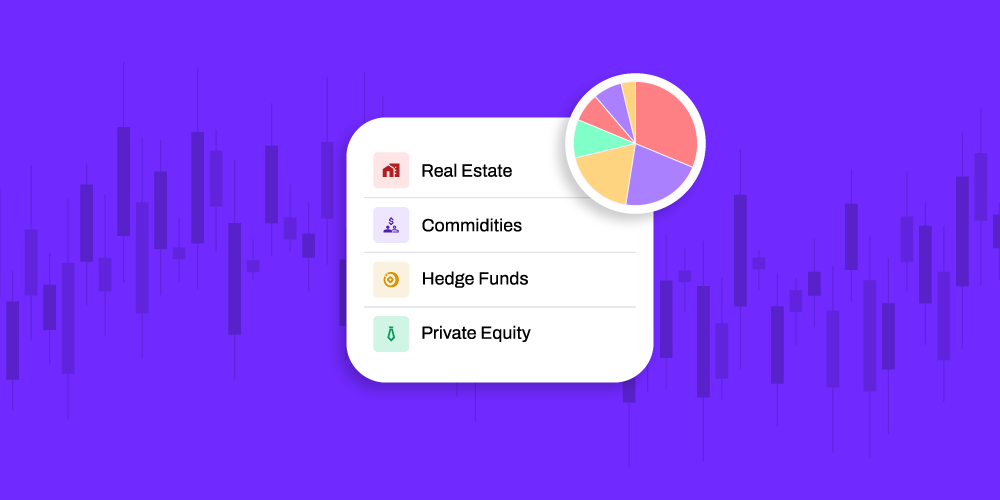Alternative investments are an excellent way to diversify your portfolio. One important gauge of success is to assess alternative investment performance.

Some alternative investments do not use the same approaches and markets as traditional investment options, such as stocks and bonds. That’s why you need to understand how to assess these investment performances to provide comparable measures.

There are many different types of alternative investments to consider, including:

• Private equity
• Private debt
• Real estate
• Commodities
• Hedge funds
• Cryptocurrencies
• Precious metals
• Timber

As you can see, the benefits of alternative investments are varied and diverse. That’s one of the reasons you need good ways to measure their performance.

Here is a closer look at 5 metrics to watch for alternative investment performance.

## 1. Return on Investment

ROI is a longstanding measure of return used widely to assess the impact of financial investments. It is one of the simplest performance metrics for alternative investments.

To calculate ROI, you calculate the amount you gained compared to the amount you invested in the asset. Take the total income for the investment over the lifetime of your investment. Divide it by the principal amount you invested. This gives you your basic ROI.

You can further refine that calculation. Take the ROI and divide it by the term of the total investment (typically in years or fractions of years). This gives you the annual percentage rate return on your investment. Side by side, the ROI and annual rate of return are helpful basic measures.

## 2. Ratios to Use for Alternative Investment Performance

There are several performance ratios investors can calculate to assess the relative values of their investments.

### Sharpe Ratio

Many ratios used for evaluation measure returns related to risk. The Sharpe ratio is one such tool, used to measure the risk-adjusted return on investment.

It’s calculated by taking the difference between the portfolio return and the risk-free rate of return. That figure is divided by the standard deviation of a portfolio’s excess return.

Because it uses standard deviations of returns as a proxy for risk, the Sharpe ratio can be misleading. It can be difficult to use on alternative investments that do not have a normal distribution of anticipated returns.

### Sortino Ratio

Many investors use the Sortino ratio in tandem with the Sharpe ratio. The Sortino ratio removes the impact of upward price movements on standard deviation measures.

The formula replaces the risk-free rate in the numerator with the required rate of return. The denominator is the distribution of returns below a targeted or required return.

With a higher Sortino rate, the higher the portfolio’s return is over the targeted return rate. It’s beneficial for analyzing highly volatile assets since it focuses on returns that are negative or below a certain set level.

### Treynor Ratio

Are you being compensated for taking extra risks in the market? The Treynor ratio measures risk placement. It measures returns that exceed what would have been earned on investment with typical market risk.

It's calculated by taking the portfolio return and subtracting the risk-free rate. While there is no investment that is entirely risk-free, treasury bill rates are typically used as a proxy.

That result is divided by beta, which is the measure of an asset’s risk compared to the market risk.

The higher the Treynor ratio, the more suitable the investment is.

### Appraisal ratio

The appraisal ratio is typically used to assess hedge funds. It compares a fund’s alpha to the portfolio’s risk specific to a stock. Alpha is the difference between an asset’s return against the benchmark return. It’s used to compare the return of a fund manager’s stock selections to the risk of those selections.

## 3. Cryptocurrency Metrics

Cryptocurrency is a newer, technology-driven alternative investment. It has markets and price volatility much like stocks and mutual funds. Yet returns are often based on supply and demand and the volume of trades. As such, the metrics used are unique, too. Here are a few metrics to keep in mind:

• Market Capitalization: In crypto, the market cap is calculated by taking the coin price and multiplying it by the total current circulating supply
• Volume 24hr: This measures the total dollar value of all transactions for particular crypto within the past 24 hours. It’s used to demonstrate liquidity -- how easy it is to buy or sell a coin
• Supply and Max Supply: Supply is the total number of circulating coins. At best, it’s an estimate as it is difficult to know which tokens are held by long-term investors. Max supply is the total amount of coins that will be in circulation. Not every coin has a fixed limit to its supply
• Exchange Flows: These measures indicate activity within a crypto exchange. Three key indicators are:
• Exchange inflow (coins transferred from a non-exchange wallet to an exchange wallet)
• Exchange outflow (coins transferred from an exchange wallet to a non-exchange wallet
• Exchange balance (Inflow minus outflow)

## 4. Public Market Equivalent (PME)

Private equity investment funds can be a challenge to measure, compared to traditional investment asset classes. The challenge is that many private equity investments are unlisted or illiquid. Providing a market-to-market comparison is challenging.

The Public Market Equivalent allows you to compare a private equity’s fund performance to that of publicly traded assets. It uses an index-return measure that factors in the irregular timing of cash flows. It compares those returns against an equivalent investment in a public market, usually a fund index like the S&P 500.

## 5. Compound Annual Growth Rate (CAGR)

The compound annual growth rate measures the average annual growth rate of an investment over time. It assumes that profits are reinvested in the asset at the end of each time period. It also smooths out returns by assuming a constant rate of growth.

While it does not reflect the actual real returns, CAGR shows the rate it could have grown if it did so at a steady rate. That means you can get a better understanding of how an investment grows over time.

To calculate the CAGR, take the end value and divide it by the beginning value. Raise that value by 1 divided by the number of years. Then subtract 1 and multiply by 100.

Having precise, accurate measures helps you determine the strength of your alternative investment performance and readjust your portfolio if necessary. It also allows you to make smarter future investment decisions that yield the best returns. If you’re ready to take the dive into alternative investments, see how Rocket Dollar can help.#### Finding and Managing Old 401(k)s

In today's fast-paced world, it's easy for individuals to lose track of their old 401(k) accounts from previous employers. For many folks, job...#### Top 9 Alternative Investments To Make With a Self-Directed IRA

Investing in alternative assets can be a great way to diversify your portfolio and potentially achieve higher returns. One way to invest in these...#### Alternatives Assets as a Hedge Against Market Downturns

When most people think of investing, they typically think of stocks, bonds, and mutual funds. However, there are also alternative investments that...